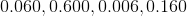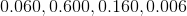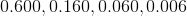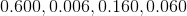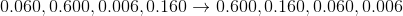## Example Questions

### Example Question #11 : How To Order Decimals From Least To Greatest Or From Greatest To Least

Which decimal is the greatest?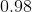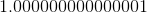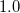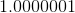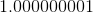Explanation:

There is ain front of most decimals exceptwhich makes the rest bigger than this decimal.

Next, the ones with less zeroes means the value is bigger and further than.

We have a decimal withzeroes, another withzeroes, and lastly withzeroes.

The one withzeroes is the greatest decimal.

That value is.

### Example Question #12 : How To Order Decimals From Least To Greatest Or From Greatest To Least

Which decimal is the SMALLEST?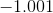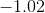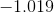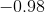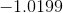Explanation:

Remember, when dealing with negative numbers, the larger the decimal, the smaller the value.

We eliminateas it is the smallest decimal value, however the greatest with the negative sign present.

By comparing to the hundredths digit,has the highest hundredths digit but is the smallest value since we have a negative sign present.is our answer.

### Example Question #101 : Decimals

Order the following from greatest to least.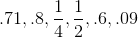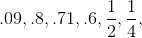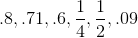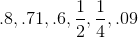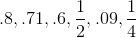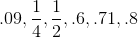Explanation: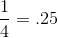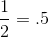List the elements from greatest to least. The greater numbers are the decimals closest to 1.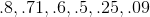### Example Question #21 : How To Order Decimals From Least To Greatest Or From Greatest To Least

Order these numbers from greatest to least: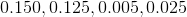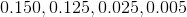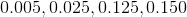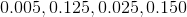Explanation:

Ordering decimals from greatest to least means we list them from the biggest number to the smallest number. When doing this, line up each of your numbers by their decimal points. We look at the tenths place first and order them according to that number. Then move on to the hundredths place and so on until each of the numbers are lined up from greatest to smallest. For this problem that would look like this: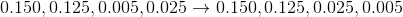### Example Question #21 : Ordering

Order these decimals from greatest to least: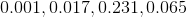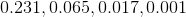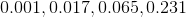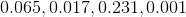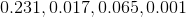Explanation:

Ordering decimals from greatest to least means we list them from the biggest number to the smallest number. When doing this, line up each of your numbers by their decimal points. We look at the tenths place first and order them according to that number. Then move on to the hundredths place and so on until each of the numbers are lined up from greatest to smallest. For this problem that would look like this: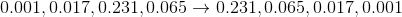### Example Question #22 : Ordering

Order these numbers from greatest to least: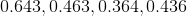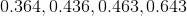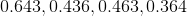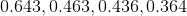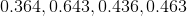Explanation:

Ordering decimals from greatest to least means we list them from the biggest number to the smallest number. When doing this, line up each of your numbers by their decimal points. We look at the tenths place first and order them according to that number. Then move on to the hundredths place and so on until each of the numbers are lined up from greatest to smallest. For this problem that would look like this: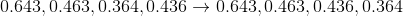### Example Question #451 : Arithmetic

Order these decimals from greatest to least: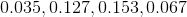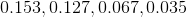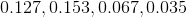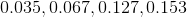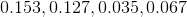Explanation:

Ordering decimals from greatest to least means we list them from the biggest number to the smallest number. When doing this, line up each of your numbers by their decimal points. We look at the tenths place first and order them according to that number. Then move on to the hundredths place and so on until each of the numbers are lined up from greatest to smallest. For this problem that would look like this: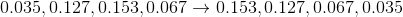### Example Question #102 : Decimals

Order these decimals from greatest to least: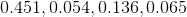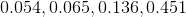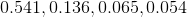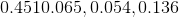Explanation:

Ordering decimals from greatest to least means we list them from the biggest number to the smallest number. When doing this, line up each of your numbers by their decimal points. We look at the tenths place first and order them according to that number. Then move on to the hundredths place and so on until each of the numbers are lined up from greatest to smallest. For this problem that would look like this: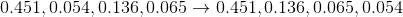### Example Question #21 : Ordering

Order these decimals from greatest to least: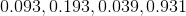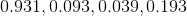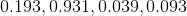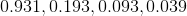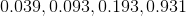Explanation:

Ordering decimals from greatest to least means we list them from the biggest number to the smallest number. When doing this, line up each of your numbers by their decimal points. We look at the tenths place first and order them according to that number. Then move on to the hundredths place and so on until each of the numbers are lined up from greatest to smallest. For this problem that would look like this: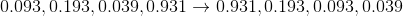### Example Question #24 : Ordering

Order these decimals from greatest to least: